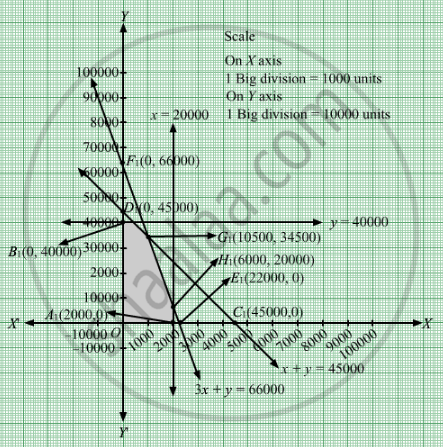# A Manufacturer of Patent Medicines is Preparing a Production Plan on Medicines, a and B.How Should the Manufacturer Schedule His Production in Order to Maximize His Profit? - Mathematics

Sum

A manufacturer of patent medicines is preparing a production plan on medicines, A and B. There are sufficient raw materials available to make 20000 bottles of A and 40000 bottles of B, but there are only 45000 bottles into which either of the medicines can be put. Further, it takes 3 hours to prepare enough material to fill 1000 bottles of A, it takes 1 hour to prepare enough material to fill 1000 bottles of B and there are 66 hours available for this operation. The profit is Rs 8 per bottle for A and Rs 7 per bottle for B. How should the manufacturer schedule his production in order to maximize his profit?

#### Solution

Let x bottles of medicine A and y bottles of medicine are prepared.
Number of bottles cannot be negative.
Therefore,

$x, y \geq 0$ According to question, the constraints are

$x \leq 20000$
$y \leq 40000$
$x + y \leq 45000$

It takes 3 hours to prepare enough material to fill 1000 bottles of A, it takes 1 hour to prepare enough material to fill 1000 bottles of B
Time taken to fill one bottle of A is $\frac{3}{1000}$ hrs and time taken by to fill one bottle of B is $\frac{1}{1000}$ hrs . Therefore, time taken to fill x bottles of A and bottles of B is $\frac{3x}{1000}$ hrs and $\frac{y}{1000}$hrs respectively.
It is given that there are 66 hours available for this operation.
$\therefore \frac{3x}{1000} + \frac{y}{1000} \leq 66$
The profit is Rs 8 per bottle for A and Rs 7 per bottle for B.   Therefore, profit gained on xbottles of medicine A and y bottles of medicine  is 8x and 7y respectively.
Total profit = Z = $8x + 7y$  which is to be maximised.
Thus, the mathematical formulat​ion of the given linear programmimg problem is
Max Z =  $8x + 7y$
subject to
$x \leq 20000$
$y \leq 40000$
$x + y \leq 45000$

$\frac{3x}{1000} + \frac{y}{1000} \leq 66 \Rightarrow 3x + y \leq 66000$
$x, y \geq 0$
First we will convert inequations into equations as follows:
x =20000, y = 40000, + y = 45000, 3x + = 66000, x = 0 and y = 0

Region represented by x  ≤ 20000:
The line x  = 20000 is the line that passes through A1(20000, 0) and is parallel to Y axis.The region to the left of the line x  = 20000 will satisfy the inequation x  ≤ 20000.

Region represented by  ≤ 40000:
The line y = 40000 is the line that passes through B1(0, 40000) and is parallel to X axis.The region below the line y  = 40000 will satisfy the inequation  ≤ 40000.

Region represented by x y ≤ 45000:
The line x + y = 45000 meets the coordinate axes at C1(45000, 0) and D1(0, 45000) respectively. By joining these points we obtain the line x + y = 45000. Clearly (0,0) satisfies the inequation x y ≤ 45000. So,the region which contains the origin represents the solution set of the inequation x y ≤ 45000.

Region represented by 3x y ≤ 66000:
The line 3x + y = 66000 meets the coordinate axes at E1(22000, 0) and $F_1 \left( 0, 66000 \right)$ respectively. By joining these points we obtain the line 3x + y = 66000. Clearly (0,0) satisfies the inequation 3x y ≤ 66000. So,the region which contains the origin represents the solution set of the inequation 3x y ≤ 66000.

Region represented by ≥ 0 and y ≥ 0:
Since, every point in the first quadrant satisfies these inequations. So, the first quadrant is the region represented by the inequations x ≥ 0, and y ≥ 0.

The feasible region determined by the system of constraints x  ≤ 20000,  ≤ 40000, x y≤ 45000, 3x y ≤ 66000, x ≥ 0 and y ≥ 0 are as follows.The corner points are O(0, 0), B1(0, 40000), G1(10500, 34500), H1(6000, 20000) and A1(20000, 0).

The values of Z at these corner points are as follows

 Corner point Z= 8x + 7y O 0 B1 280000 G1 325500 H1 188000 A1 160000

The maximum value of Z is 325500 which is attained at G1(10500, 34500).
Thus, the maximum profit is Rs 325500 obtained when 10500 bottles of A and 34500 bottles of B were manufactured.
Concept: Graphical Method of Solving Linear Programming Problems
Is there an error in this question or solution?

#### APPEARS IN

RD Sharma Class 12 Maths
Chapter 30 Linear programming
Exercise 30.4 | Q 21 | Page 53